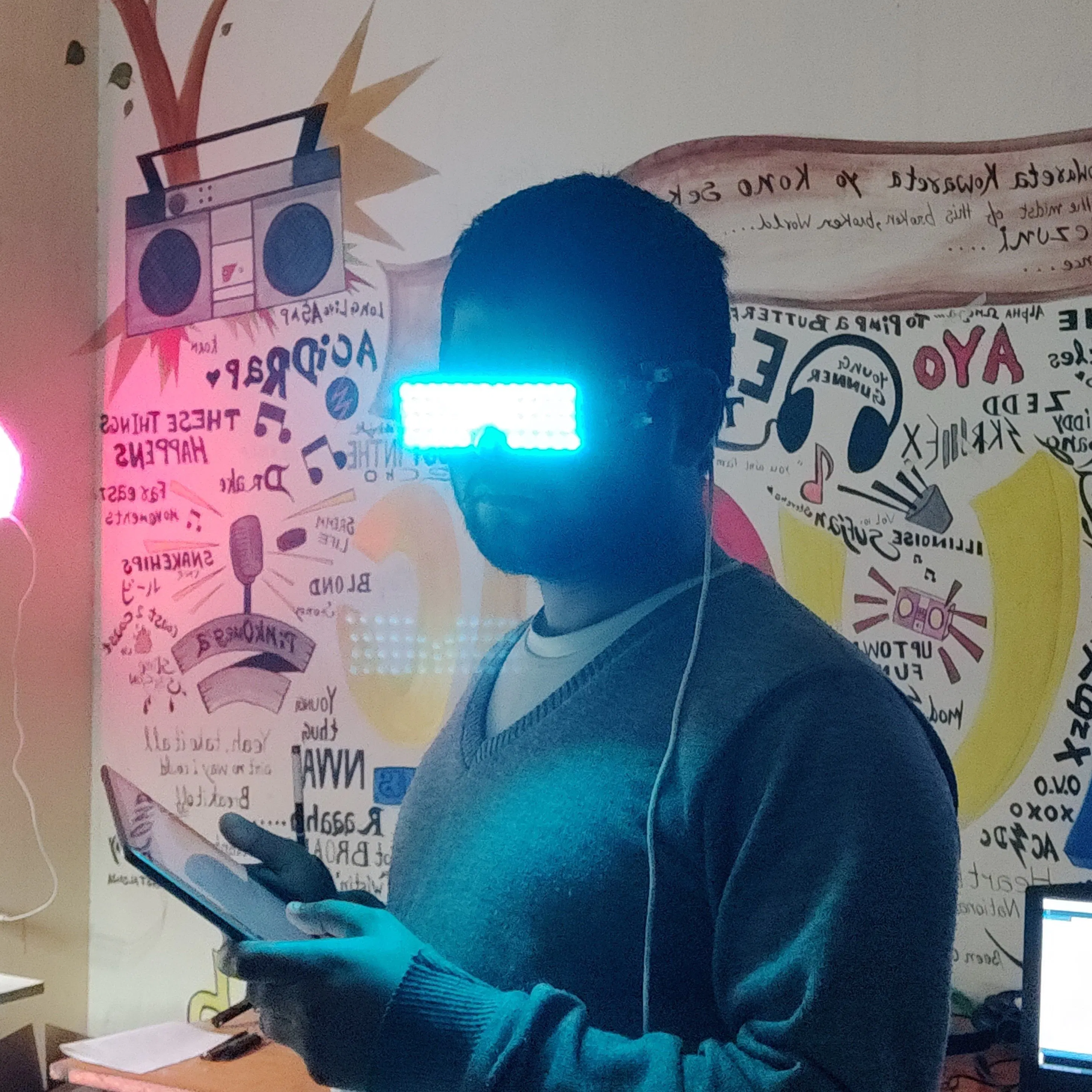Published © GPL3+

# Arduino Atari Punk Synth

A DIY version of Atari Punk Synth with minimal stuff.

BeginnerFull instructions provided24 minutes2,276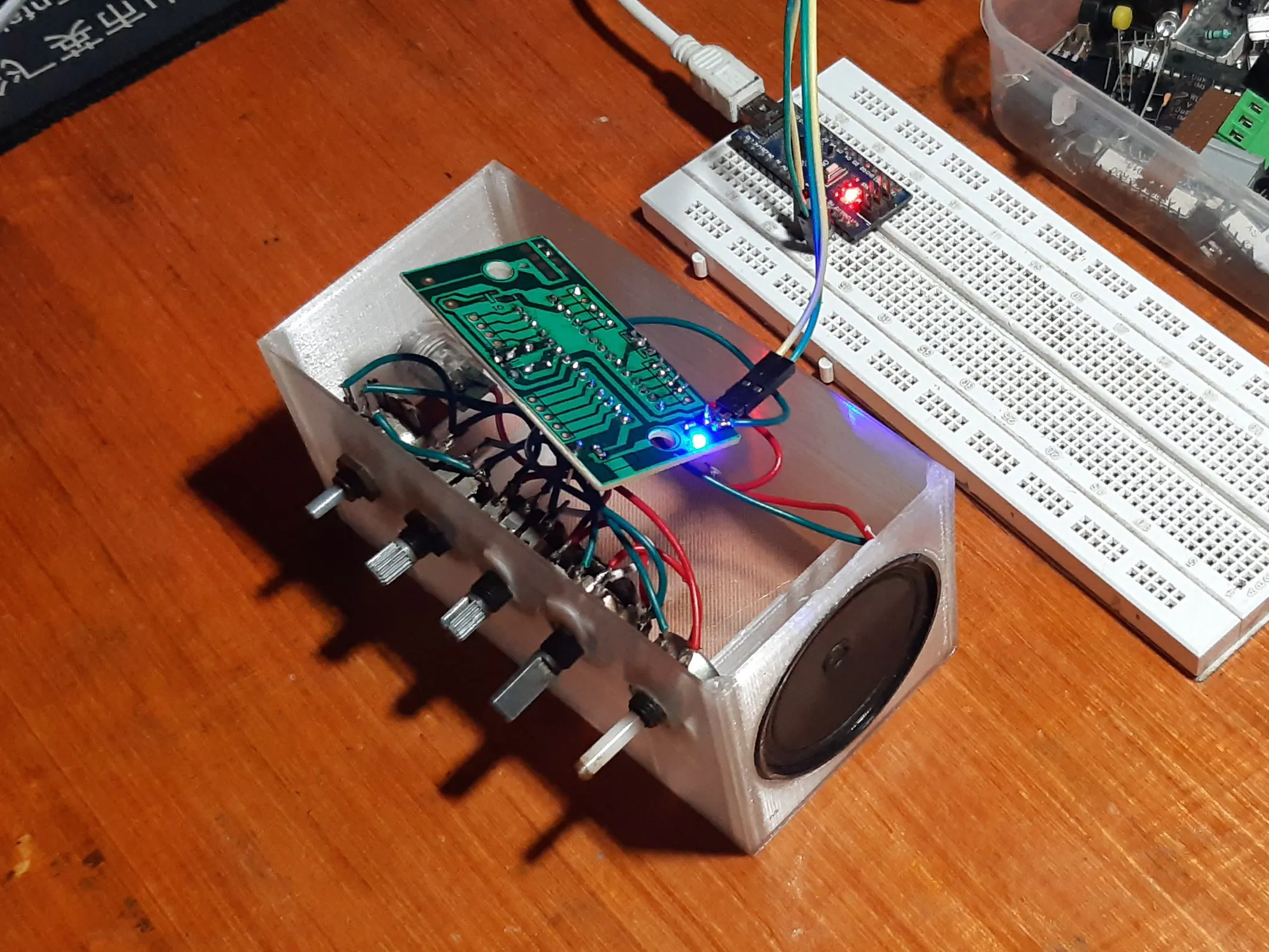## Things used in this project

### Hardware components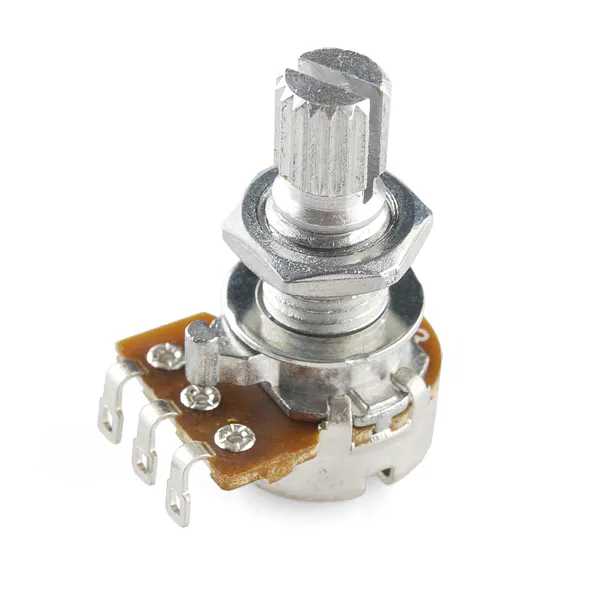Rotary potentiometer (generic)
×5UTSOURCE Electronic Parts everything above can be found here for a low price
×1Arduino UNO
×1JLCPCB Customized PCB
×1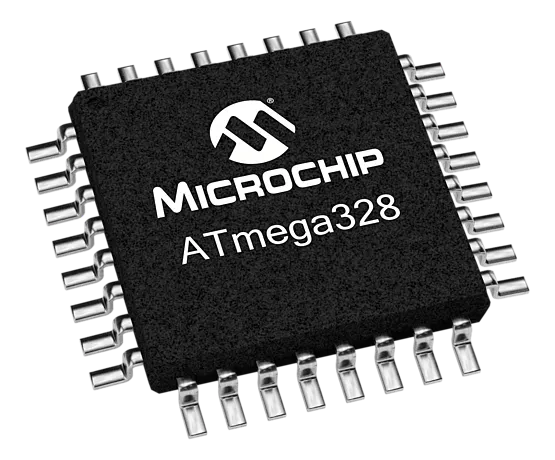Microchip Technology ATmega328
×1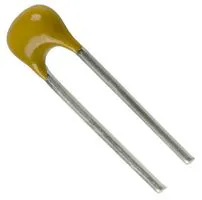Capacitor 22 pF
×2
 16mhz crystal
×1

### Software apps and online servicesArduino IDE

### Hand tools and fabrication machines3D Printer (generic)

Read more

## Custom parts and enclosures

### base body

Sketchfab still processing.

## Code

### code

C/C++
```#include <MozziGuts.h>
#include <Oscil.h> // oscillator
#include <tables/cos2048_int8.h> // table for Oscils to play
#include <Smooth.h>
#include <AutoMap.h> // maps unpredictable inputs to a range

// int freqVal;

// desired carrier frequency max and min, for AutoMap
const int MIN_CARRIER_FREQ = 22;
const int MAX_CARRIER_FREQ = 440;

const int MIN = 1;
const int MAX = 10;

const int MIN_2 = 1;
const int MAX_2 = 15;

// desired intensity max and min, for AutoMap, note they're inverted for reverse dynamics
const int MIN_INTENSITY = 700;
const int MAX_INTENSITY = 10;

// desired mod speed max and min, for AutoMap, note they're inverted for reverse dynamics
const int MIN_MOD_SPEED = 10000;
const int MAX_MOD_SPEED = 1;

AutoMap kMapCarrierFreq(0,1023,MIN_CARRIER_FREQ,MAX_CARRIER_FREQ);
AutoMap kMapIntensity(0,1023,MIN_INTENSITY,MAX_INTENSITY);
AutoMap kMapModSpeed(0,1023,MIN_MOD_SPEED,MAX_MOD_SPEED);
AutoMap mapThis(0,1023,MIN,MAX);
AutoMap mapThisToo(0,1023,MIN_2,MAX_2);

const int KNOB_PIN = 0; // set the input for the knob to analog pin 0
const int LDR1_PIN=1; // set the analog input for fm_intensity to pin 1
const int LDR2_PIN=2; // set the analog input for mod rate to pin 2
const int LDR3_PIN=4;
const int LDR4_PIN=3;

Oscil<COS2048_NUM_CELLS, AUDIO_RATE> aCarrier(COS2048_DATA);
Oscil<COS2048_NUM_CELLS, AUDIO_RATE> aModulator(COS2048_DATA);
Oscil<COS2048_NUM_CELLS, CONTROL_RATE> kIntensityMod(COS2048_DATA);

int mod_ratio = 5; // brightness (harmonics)
long fm_intensity; // carries control info from updateControl to updateAudio

// smoothing for intensity to remove clicks on transitions
float smoothness = 0.95f;
Smooth <long> aSmoothIntensity(smoothness);

void setup(){
//  Serial.begin(115200); // set up the Serial output so we can look at the light level
startMozzi(); // :))
}

void updateControl(){

//  freqVal = map(LDR3_PIN, 0, 1023, 1, 100);

int freqVal = mozziAnalogRead(LDR3_PIN); // value is 0-1023
int FRQ = mapThis(freqVal);

int knob2 = mozziAnalogRead(LDR4_PIN); // value is 0-1023
int knob2Val = mapThis(knob2);

// read the knob
int knob_value = mozziAnalogRead(KNOB_PIN); // value is 0-1023

// map the knob to carrier frequency
int carrier_freq = kMapCarrierFreq(knob_value);

//calculate the modulation frequency to stay in ratio
int mod_freq = carrier_freq * mod_ratio * FRQ;

// set the FM oscillator frequencies
aCarrier.setFreq(carrier_freq);
aModulator.setFreq(mod_freq);

// read the light dependent resistor on the width Analog input pin
int LDR1_value= mozziAnalogRead(LDR1_PIN); // value is 0-1023
// print the value to the Serial monitor for debugging
//Serial.print("LDR1 = ");
// Serial.print(LDR1_value);
// Serial.print("\t"); // prints a tab

int LDR1_calibrated = kMapIntensity(LDR1_value);
// Serial.print("LDR1_calibrated = ");
// Serial.print(LDR1_calibrated);
//  Serial.print("\t"); // prints a tab

// calculate the fm_intensity
fm_intensity = ((long)LDR1_calibrated * knob2Val * (kIntensityMod.next()+128))>>8; // shift back to range after 8 bit multiply
//  Serial.print("fm_intensity = ");
// Serial.print(fm_intensity);
//  Serial.print("\t"); // prints a tab

// read the light dependent resistor on the speed Analog input pin
int LDR2_value= mozziAnalogRead(LDR2_PIN); // value is 0-1023
//  Serial.print("LDR2 = ");
//  Serial.print(LDR2_value);
//  Serial.print("\t"); // prints a tab

// use a float here for low frequencies
float mod_speed = (float)kMapModSpeed(LDR2_value)/1000;
//Serial.print("   mod_speed = ");
// Serial.print(mod_speed);
kIntensityMod.setFreq(mod_speed);

// Serial.println(); // finally, print a carraige return for the next line of debugging info
}

int updateAudio(){
long modulation = aSmoothIntensity.next(fm_intensity) * aModulator.next();
return aCarrier.phMod(modulation);
}

void loop(){
audioHook();
}
```

## Credits

### Arnov Sharma

91 projects • 141 followers
Just your average MAKER